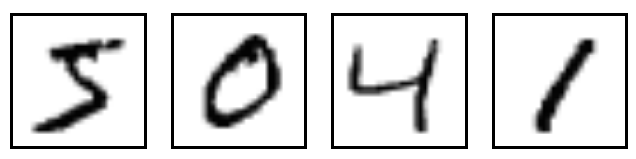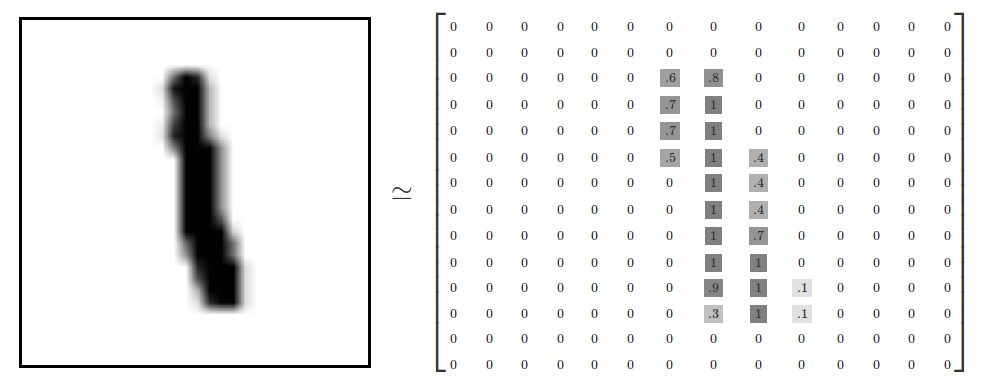TensorFlow allows us to perform machine learning operations on huge matricies with large efficiency. It can also easily distribute this processing across CPU cores, GPU cores, or even multiple devices like multiple GPUs. Tensor, in TensorFlow is an array-like object, and, similar to an array it can hold matrix, vector, and even a scalar. In this tutorial we’ll work with MNIST dataset. MNIST is a simple computer vision dataset. It consists of images of handwritten digits like the image below. We will then train a deep neural network on the training set using TensorFlow and make predictions on a test set.Resources:

Contents

## Understanding and Importing the MNIST Data

We’re going to be working first with the MNIST dataset, which is a dataset that contains 60,000 training samples and 10,000 testing samples of hand-written and labeled digits, 0 through 9, so ten total “classes.”

The MNIST dataset has the images (see example above), which we’ll be working with as purely black and white, thresholded, images, of size 28 x 28, or 784 pixels total.Our features will be the pixel values for each pixel, thresholded. Either the pixel is “blank” (nothing there, a 0), or there is something there (1). Those are our features. We’re going to attempt to just use this extremely rudimentary data, and predict the number we’re looking at (a 0,1,2,3,4,5,6,7,8, or 9).

``````import tensorflow as tf

from tensorflow.examples.tutorials.mnist import input_data
mnist = input_data.read_data_sets("/tmp/data/", one_hot = True)
``````
``````Extracting /tmp/data/train-images-idx3-ubyte.gz
Extracting /tmp/data/train-labels-idx1-ubyte.gz
Extracting /tmp/data/t10k-images-idx3-ubyte.gz
Extracting /tmp/data/t10k-labels-idx1-ubyte.gz
``````

The MNIST data is split into three parts: 55,000 data points of training data (mnist.train), 10,000 points of test data (mnist.test), and 5,000 points of validation data (mnist.validation).

For the purposes of this tutorial, we’re going to want our labels as “one-hot vectors”. A one-hot vector is a vector which is 0 in most dimensions, and 1 in a single dimension. In this case, the nth digit will be represented as a vector which is 1 in the nth dimension. For example, 3 would be [0,0,0,1,0,0,0,0,0,0]. Consequently, mnist.train.labels is a [55000, 10] array of floats.

## Setting up the Computation Model

In case of neural network,

We have feature data, X, value in each pixel, weights (w), and thresholds or biases (t).

TensorFlow works by first defining and describing our model in abstract, and then, when we are ready, we make it a reality in the session. The description of the model is what is known as your “Computation Graph” in TensorFlow terms. Here is the algorithm:

• We begin by specifying how many nodes each hidden layer will have, how many classes our dataset has, and what our batch size will be.
• First, we take our input data, and we need to send it to hidden layer 1.
• We weight the input data, and send it to layer 1, where it will undergo the activation function,
• The neuron can decide whether or not to output data to either output layer, or another hidden layer.
• We will have three hidden layers in this example, making this a Deep Neural Network.
• From the output we get, we will start training.
``````# defining number of hidden layers, nodes in each hidden layer
n_nodes_hl1 = 500
n_nodes_hl2 = 500
n_nodes_hl3 = 500
n_classes = 10
batch_size = 100
``````
``````# placeholders for variables x and y
x = tf.placeholder('float', [None, 784])
y = tf.placeholder('float')
``````

We have used [None,784] as a 2nd parameter in the first placeholder. This is an optional parameter. It can be useful, however, to be explicit like this. We’re now complete with our constants and starting values. Now we can actually build the Neural Network Model

``````def neural_network_model(data):

"""Layers definitions"""
hidden_1_layer = {'weights':tf.Variable(tf.random_normal([784, n_nodes_hl1])),
'biases':tf.Variable(tf.random_normal([n_nodes_hl1])) }

hidden_2_layer = {'weights':tf.Variable(tf.random_normal([n_nodes_hl1, n_nodes_hl2])),
'biases':tf.Variable(tf.random_normal([n_nodes_hl2])) }

hidden_3_layer = {'weights':tf.Variable(tf.random_normal([n_nodes_hl2, n_nodes_hl3])),
'biases':tf.Variable(tf.random_normal([n_nodes_hl3])) }

output_layer = {'weights':tf.Variable(tf.random_normal([n_nodes_hl3, n_classes])),
'biases':tf.Variable(tf.random_normal([n_classes])) }

"""Feed Forward"""
# input_data*weights + biases
# relu (rectified linear) activation function
# layer 1
l1 = tf.nn.relu(l1)

l2 = tf.nn.relu(l2)

l3 = tf.nn.relu(l3)

return output
``````

The bias is a value that is added to our sums, before being passed through the activation function, not to be confused with a bias node, which is just a node that is always on. The purpose of the bias here is mainly to handle for scenarios where all neurons fired a 0 into the layer. A bias makes it possible that a neuron still fires out of that layer. A bias is as unique as the weights, and will need to be optimized too.

## Training the Model

Under a new function, train_neural_network, we will pass our output data.

• We then produce a prediction based on the output of that data through our neural_network_model.
• Next, we create a cost variable. This measures how wrong we are, and is the variable we desire to minimize by manipulating our weights. The cost function is synonymous with a loss function.
• To optimize our cost, we will use the AdamOptimizer, which is a popular optimizer along with others like Stochastic Gradient Descent and AdaGrad, for example.
• Within AdamOptimizer(), we can optionally specify the learning_rate as a parameter. The default is 0.001, which is fine for most circumstances.
• Now that we have these things defined, we begin the session.
``````def train_neural_network(x):
# predictions from one feedforward epoch
prediction = neural_network_model(x)

# Minimizing the cost
cost = tf.reduce_mean( tf.nn.softmax_cross_entropy_with_logits(logits=prediction, labels=y) )

# total number of epochs
hm_epochs = 10

# Begin the session
with tf.Session() as sess:
sess.run(tf.global_variables_initializer())
for epoch in range(hm_epochs):
epoch_loss = 0
for _ in range(int(mnist.train.num_examples/batch_size)):
epoch_x, epoch_y = mnist.train.next_batch(batch_size)
_, c = sess.run([optimizer, cost], feed_dict = {x: epoch_x, y: epoch_y})
epoch_loss += c
print('Epoch', epoch, 'completed out of',hm_epochs,'loss:',epoch_loss)

correct = tf.equal(tf.argmax(prediction, 1), tf.argmax(y, 1))

accuracy = tf.reduce_mean(tf.cast(correct, 'float'))
print('Accuracy:',accuracy.eval({x:mnist.test.images, y:mnist.test.labels}))

train_neural_network(x)

``````
``````Epoch 0 completed out of 10 loss: 1794357.3494
Epoch 1 completed out of 10 loss: 404222.131447
Epoch 2 completed out of 10 loss: 220933.089828
Epoch 3 completed out of 10 loss: 130508.189908
Epoch 4 completed out of 10 loss: 82744.7880272
Epoch 5 completed out of 10 loss: 51979.5030085
Epoch 6 completed out of 10 loss: 34854.3968684
Epoch 7 completed out of 10 loss: 27911.6349728
Epoch 8 completed out of 10 loss: 21536.7758809
Epoch 9 completed out of 10 loss: 18292.1778653
Accuracy: 0.9525
``````

## Accuracy and Conclusion

So our implementation of deep neural networks give an accuracy of 95%. Consider that the only information we gave to our network was pixel values, that’s it. We did not tell it about looking for patterns, or how to tell a 4 from a 9, or a 1 from a 8. The network simply figured it out with an inner model, based purely on pixel values to start, and achieved 95% accuracy in just 10 epochs. 95% accuracy, however, isn’t considered the best and most of the applications of neural networks work on over 99% accuracy. In next coming posts I will use this same dataset with more complex neural networks such as Convolution Neural Networks and Recurring Neural Networks to improve the accuracy.